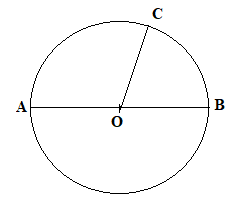Courses
Courses for Kids
Free study material
Free LIVE classes
MoreLIVE
Join Vedantu’s FREE Mastercalss

# The relation between radius and diameter of a circle is . A. Radius = 2$\times {\text{ diameter}}$ B. Radius = diameter$\div 2$ C. Diameter = radius $\div 2$ D. Diameter = 2 $\times {\text{ radius}}$ .Verified
360.6k+ views
Hint: In this question, we have to find the relation between the radius and diameter of a circle. The relation between the radius and the diameter of a circle is obtained once we draw the diagram of a circle. Draw a line passing through the centre and touching the circumference of the circle and then measure the length of diameter and radius.

Complete step-by-step solution -
In the question we have to find the relationship between the radius and the diameter of the circle.
So first of all we will draw the diagram of the circle.We know that the distance between the centre of the circle and its circumference is known as radius of circle.
$\therefore$ OC, OB and OA are radii of the given circle.
And we know that diameter is a line which passes through the centre and touches the circumference on both sides of the circle.
$\therefore$ AB is the diameter of the circle.
But AB = OA+OB.
$\because$ OA =OB
Therefore, AB = $2 \times$ OA
Diameter = 2$\times$ radius.
So option D is correct.

Note: In this type of question, you should remember the definition of radius of circle and diameter of circle. After this, draw the diagram of the circle indicating the radius and centre of the circle. Now use the definition of radius and diameter of the circle to find the relationship between the radius and diameter.
Last updated date: 26th Sep 2023
Total views: 360.6k
Views today: 4.60k